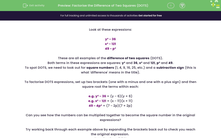# Factorise the Difference of Two Squares (DOTS)

In this worksheet, students will practise identifying expressions which use DOTS, and then factorise or expand pairs of brackets to create alternative expressions of the same value.Key stage:  KS 4

Year:  GCSE

GCSE Subjects:   Maths

GCSE Boards:   Pearson Edexcel, OCR, Eduqas, AQA,

Curriculum topic:   Algebra

Curriculum subtopic:   Notation, Vocabulary and Manipulation Algebraic Expressions

Popular topics:   Square Numbers worksheets

Difficulty level:#### Worksheet Overview

Look at these expressions:

y² - 36

x² - 121

49 - p²

These are all examples of the difference of two squares (DOTS).

Both terms in these expressions are squares:  and 36 and 121, and 49.

To spot DOTS, we need to look out for square numbers (1, 4, 9, 16, 25, etc.) and a subtraction sign (this is what 'difference' means in the title).

To factorise DOTS expressions, set up two brackets (one with a minus and one with a plus sign) and then square root the terms within each:

e.g. y² - 36 = (y - 6)(y + 6)

e.g. x² - 121 = (x - 11)(x + 11)

49 - 4p² = (7 - 2p)(7 + 2p)

Can you see how the numbers can be multiplied together to become the square number in the original expressions?

Try working back through each example above by expanding the brackets back out to check you reach the original expression.

It works, doesn't it?!

In this activity, we will practise identifying expressions which use DOTS and then factorise or expand pairs of brackets to create alternative expressions of the same value.

### What is EdPlace?

We're your National Curriculum aligned online education content provider helping each child succeed in English, maths and science from year 1 to GCSE. With an EdPlace account you’ll be able to track and measure progress, helping each child achieve their best. We build confidence and attainment by personalising each child’s learning at a level that suits them.

Get started